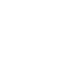# SAT Question of the Day (ACT too): Oct. 13, 2013

If you are reading this in an email you received from me, do not click the link to sat.collegeboard.org below. Use the link to my website that is farther down on the email. If you are seeing this in my blog, do the SAT Question of the Day by clicking on this link:

http://sat.collegeboard.org/practice/sat-question-of-the-day?questionId=20131013&oq=1 (This link takes you to today’s question. If you use my archive, you will see the question related to my SAT explanation for that date.)

The answer is C.  You could certainly solve the problem using the College Board’s explanation but there are sure easier ways to do it.  I did it two different ways in less time than it took me to read their explanation!

Anytime I see a question that says “what is the value of x” or something similar, I know Pillar V: The Answer Is On the Page (Video #1) is going to pay off.  This question asks “how many students,” so, I know one of the answers has to be the right number of students.  I used the answers.  Let’s begin with Answer C because if it is wrong, I know whether it is too big or too small and can eliminate two other answers at the same time.  I can eliminate 3 answers for the price of trying 1!  If 7 students (Answer C) belong to both clubs, they represent 14 memberships.  Adding those 14 to 13 students who are members of only one club and I have a total of 27 members.  Bingo!  That’s what the question said, “math club has 15 members and the chess club has 12 members:” a total of 27.  All done.

Let’s see what would have happened had I used a different answer.  Answer B is 6.  So, if 6 belonged to both clubs, that would be 12 members and the 13 who are members in one club is a total of 25 members which is too small.  That sure is easy.  Let’s try another way to do the question.

The second way to do it is just think about what is going on.  I have a total of 27 members and 13 of them are only in one club.  When I subtract 27-13, that leaves 14 memberships that are accounted for by students in two clubs.  That means they each have to be counted twice!  Simply divide 14 by 2.  The answer is 7.

I can check my work by calculating 7 students who are members twice is 14 plus 13 students who are members once is 27.  That works.

Why do the test writers make this game so hard?  It simply isn’t as bad as they make it seem.  Gosh, it’s fun to beat them at their own game!

Let’s see what the ACT folks have for us today.

Another math question, this will be fun.

The answer is G.  These guys have the same affliction as the SAT folks.  They both are infected by the “let’s see how complicated we can make the explanation” disease.  Go ahead and read how they did it and if you follow it, you probably didn’t need their help in the first place.  Congratulations.

I just added the two equations and got 3x + 3y = 6.  Divide both sides by 3 and you get x+y=2.  All done.

Do you see why 3x+3y all divided by 3 is x+y?  You can factor so that 3x+3y=3(x+y).  Then the 3’s cancel when you divide 3(x+y) by 3.

What can you learn from today’s Question of the Day from both the SAT and ACT folks?  If they can make the explanation harder to understand than the question, they’ll do it!  All kidding aside, what is really important is for you to realize there are always a number of ways to do each math question.  If you are comfortable with doing the math, good for you and that’s the way you should do the question.  However, there are wizardly ways that are often much faster.  The moral of the story is to practice and figure out which methods work best for you.  That way when you take the test, you’ll be at your most efficient and raise your score.

If you are taking the PSAT this week, be sure you work on your skills and strategies for taking the test a little bit each day.  Doing my blog is a good start.  After dinner the night before the test, do some social studies or science homework.  Don’t worry about the test anymore.  You’ll be fine.

The SAT & ACT Wizard